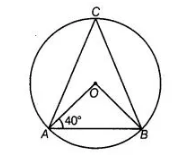# In figure, if ∠OAB = 40°,`
Question:

In figure, if ∠OAB = 40°, then ∠ACB is equal to From Eq. (i) ∠ACB = ∠ADB = 70°(a) 50°

(b) 40°

(c) 60°

(d) 70°

Solution:

(a)

In ΔQAB, OA = OB [both are the radius of a circle]

∠OAB = ∠OBA => ∠OBA = 40°

[angles opposite to equal sides are equal] Also, ∠AOB + ∠OBA + ∠BAO = 180°

[by angle sum property of a triangle]

∠AOB + 40° + 40° = 180°

=> ∠AOB = 180° – 80° = 100°

We know that, in a circle, the angle subtended by an arc at the centre is twice the angle subtended by it at the remaining part of the circle.

∠AOB = 2 ∠ACB => 100° =2 ∠ACB

∠ACB = 100°/2 = 50°